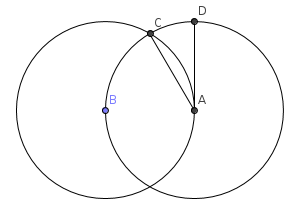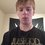So I was trying to derive a way to approximate $\pi$ using my compass and straightedge, and then use algebra. I began by creating a $30$ degree angle:So $CAD$ is $30$ degrees. Then, I imagined bisecting this angle into infinity. Notice if we draw a segment from $C$ to $D$, we get an isosceles. We can calculate for this length using the law of cosines: $x = \sqrt{2-2\cos(\frac{30}{2r}})$, where $r$ is a reiteration (another bisection). So, if I am correct in my assumption, $\pi$ should be about $x \cdot n$, if $n$ are the number of divisions in the circle. We can find $n$ easily enough: $n = 24 \cdot 2^{r-2}$. we know this becuase, as we bisect, we get a table of values: $\left \{ 24, 48, 96, \ldots \right \}$. Likewise, we find $\theta$ thusly: $\theta = \frac{30}{2^{r}}$. This table of values is found by dividing 360 by $n$: $\left \{ 30, 15, 7.5, ... \right \}$. So to find $x$, we use the law of cosine, take the square, and multiply by n. This gives me the equation: $\pi = \sqrt{2- 2 \cdot \cos \dfrac{30}{2^{r}}} \cdot 24 \cdot 2^{r-2}$ This makes sense from the way I constructed it, but not here: $\lim_{r \rightarrow \infty} \sqrt{2- 2 \cdot \cos \frac{30}{2^{r}}} \cdot 24 \cdot 2^{r-2}$

This becomes: $\lim_{r \rightarrow \infty} \sqrt{2- 2 \cdot \cos(30 \cdot 0)} \cdot 24 \cdot 2^{r-2} = 0$ Obviously, $\pi \neq 0$. So, I tested with two values of $r$ that my calculator could handle. For $r =17$, $\pi = 3.14159265358$ (correct to 11 decimal places.) However, at $r = 18$, $\pi = 3.1415$ (correct to only 4 decimal places.) So why does this equation get close to $\pi$, as it is supposed to, and then stop, and then appear to approach zero? Thanks for the help, I am not all that great at math, and would really appreciate it!Note by Drex Beckman
5 years, 1 month ago

This discussion board is a place to discuss our Daily Challenges and the math and science related to those challenges. Explanations are more than just a solution — they should explain the steps and thinking strategies that you used to obtain the solution. Comments should further the discussion of math and science.

When posting on Brilliant:

• Use the emojis to react to an explanation, whether you're congratulating a job well done , or just really confused .
• Ask specific questions about the challenge or the steps in somebody's explanation. Well-posed questions can add a lot to the discussion, but posting "I don't understand!" doesn't help anyone.
• Try to contribute something new to the discussion, whether it is an extension, generalization or other idea related to the challenge.

MarkdownAppears as
*italics* or _italics_ italics
**bold** or __bold__ bold
- bulleted- list
• bulleted
• list
1. numbered2. list
1. numbered
2. list
Note: you must add a full line of space before and after lists for them to show up correctly
paragraph 1paragraph 2

paragraph 1

paragraph 2

[example link](https://brilliant.org)example link
> This is a quote
This is a quote
    # I indented these lines
# 4 spaces, and now they show
# up as a code block.

print "hello world"
# I indented these lines
# 4 spaces, and now they show
# up as a code block.

print "hello world"
MathAppears as
Remember to wrap math in $$ ... $$ or $ ... $ to ensure proper formatting.
2 \times 3 $2 \times 3$
2^{34} $2^{34}$
a_{i-1} $a_{i-1}$
\frac{2}{3} $\frac{2}{3}$
\sqrt{2} $\sqrt{2}$
\sum_{i=1}^3 $\sum_{i=1}^3$
\sin \theta $\sin \theta$
\boxed{123} $\boxed{123}$

Sort by:

Extremely interesting! How are you saying that limit is 0? (Hint: It's an indeterminate form, ($0 \cdot \infty$))
The correct limit is $\pi$, just like you wanted.

- 5 years, 1 month ago

Well, it seemed like as r approached infinity, the cosine would approach 0. of course, cos(0) = 1 and so we would get $0 * 24 * 2^{r-2}$. Since the limit is $\pi$, is there something wrong with my calculations? Since the precision seemed to degrade for higher r's. Thanks, I don't have the experience of ever learning limits in school, so I do not realize there is such a thing as indeterminate forms, but I was unsure what to do with the $0 * \infty$ case. I just assumed for any number, you would get zero. Thanks for the help! :)

- 5 years, 1 month ago

Oh okay, I'll try to explain then. Suppose you had two functions, $f(x)$ and $g(x)$,
$\lim_{x \to \infty} f(x) = \infty$ and $\lim_{x \to \infty} g(x) = 0$. Now what is $\lim_{x \to \infty} f(x)\cdot g(x)$?

If you think about it, you really can't say, because it could be $0$ or $\infty$ or some value in between.
Why? It depends on the functions $f(x)$ and $g(x)$ themselves.
Let me give you some examples. Let $L = \lim_{x \to \infty} f(x)\cdot g(x)$.

1. $f(x) = x$ and $g(x) = \frac{1}{x} \Rightarrow L = 1$ as $f(x)g(x) = 1$ always.
2. $f(x) = x^2$ and $g(x) = \frac{1}{x} \Rightarrow L = \infty$ as $f(x)g(x) = x$ always.
3. $f(x) = x$ and $g(x) = \frac{1}{x^2} \Rightarrow L = 0$ as $f(x)g(x) = \frac{1}{x}$ always.
4. $f(x) = 5x$ and $g(x) = \frac{1}{x} \Rightarrow L = 5$ as $f(x)g(x) = 5$ always.

So, we've seen that the limit can be anything really. This is why we call $0 \cdot \infty$ an indeterminate form, it can 'evaluate' to anything.

Now, to your question, how do we evaluate $\lim_{r \rightarrow \infty} \sqrt{2- 2 \cdot \cos \frac{30}{2^{r}}} \cdot 24 \cdot 2^{r-2}$?
Here, $f(x) = 24 \cdot 2^{r - 2}$ and $g(x) = \sqrt{2 - 2 \cdot \cos \frac{30}{2^r}}$ (Understand why.)
So what is L, here?
(Convert $30$ to $\frac{\pi}{6}$ radians)
L = $\lim_{r \rightarrow \infty} \sqrt{2- 2 \cdot \cos \frac{\pi}{6 \cdot 2^{r}}} \cdot 6 \cdot 2^{r}$
Let $t = \dfrac{1}{6\cdot 2^r}$.
L = $\lim_{t \rightarrow 0} \dfrac{\sqrt{2- 2 \cdot \cos \pi t }}{t} = \lim_{t \rightarrow 0} \dfrac{\sqrt{2}\sqrt{1 - \cos \pi t }}{t} = \lim_{t \rightarrow 0} \dfrac{\sqrt{2}\sqrt{2 \sin^2 \frac{\pi t}{2}}}{t} = \lim_{t \rightarrow 0} \dfrac{2 sin \frac{\pi t}{2}}{t} = \pi$

I used the well-known facts that $1 - \cos 2x = 2 \sin^2 x$ and $\lim_{x \to 0} \frac{\sin x}{x} = 1$.

- 5 years, 1 month ago

Well explained.

It's a very common misconception to "Let $n \rightarrow \infty$ in a specific portion of the expression, while ignoring the rest of it".

Staff - 5 years, 1 month ago

Thanks a lot, I think I understand it now! Very clear explanation, also! +1

- 5 years, 1 month ago

Thanks! Also, the reason your calculator drops off in precision is because of rounding off errors and approximate values for the cosines and sines.

- 5 years, 1 month ago

Right, that was what I suspected, but I put a bit too much faith in the arbitrary precision calculations, I guess. xD

- 5 years, 1 month ago